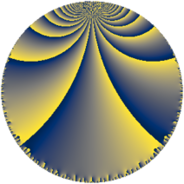# Properties

 Label 76.6.kLevel $76$ Weight $6$ Character orbit 76.k Rep. character $\chi_{76}(3,\cdot)$ Character field $\Q(\zeta_{18})$ Dimension $288$ Newform subspaces $1$ Sturm bound $60$ Trace bound $0$

# Related objects

## Defining parameters

 Level: $$N$$ $$=$$ $$76 = 2^{2} \cdot 19$$ Weight: $$k$$ $$=$$ $$6$$ Character orbit: $$[\chi]$$ $$=$$ 76.k (of order $$18$$ and degree $$6$$) Character conductor: $$\operatorname{cond}(\chi)$$ $$=$$ $$76$$ Character field: $$\Q(\zeta_{18})$$ Newform subspaces: $$1$$ Sturm bound: $$60$$ Trace bound: $$0$$

## Dimensions

The following table gives the dimensions of various subspaces of $$M_{6}(76, [\chi])$$.

Total New Old
Modular forms 312 312 0
Cusp forms 288 288 0
Eisenstein series 24 24 0

## Trace form

 $$288q - 6q^{2} + 24q^{4} - 12q^{5} + 264q^{6} - 9q^{8} + 54q^{9} + O(q^{10})$$ $$288q - 6q^{2} + 24q^{4} - 12q^{5} + 264q^{6} - 9q^{8} + 54q^{9} - 657q^{10} - 9q^{12} + 336q^{13} - 2163q^{14} + 1824q^{16} - 12q^{17} - 10242q^{20} - 7842q^{21} - 102q^{22} - 11856q^{24} - 12q^{25} + 1317q^{26} + 6660q^{28} - 12q^{29} - 40692q^{30} - 3351q^{32} - 8604q^{33} - 15756q^{34} + 81783q^{36} + 50400q^{38} + 39246q^{40} - 29082q^{41} - 123081q^{42} - 39651q^{44} - 6q^{45} - 112554q^{46} - 68403q^{48} + 259302q^{49} + 154188q^{50} - 36867q^{52} - 26280q^{53} + 172605q^{54} - 12q^{57} + 229740q^{58} - 136062q^{60} + 169296q^{61} + 35076q^{62} + 63795q^{64} - 189918q^{65} + 73644q^{66} + 59706q^{68} - 200898q^{69} - 177831q^{70} - 188502q^{72} + 397428q^{73} - 530703q^{74} - 53022q^{76} + 159804q^{77} - 618237q^{78} + 18045q^{80} - 110160q^{81} + 155859q^{82} + 733851q^{84} - 645108q^{85} + 616836q^{86} + 598383q^{88} - 226320q^{89} + 546366q^{90} - 754308q^{92} + 558942q^{93} - 454530q^{96} - 177198q^{97} - 76791q^{98} + O(q^{100})$$

## Decomposition of $$S_{6}^{\mathrm{new}}(76, [\chi])$$ into newform subspaces

Label Dim. $$A$$ Field CM Traces $q$-expansion
$$a_2$$ $$a_3$$ $$a_5$$ $$a_7$$
76.6.k.a $$288$$ $$12.189$$ None $$-6$$ $$0$$ $$-12$$ $$0$$